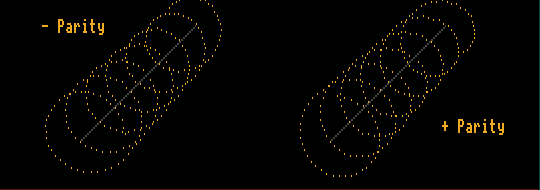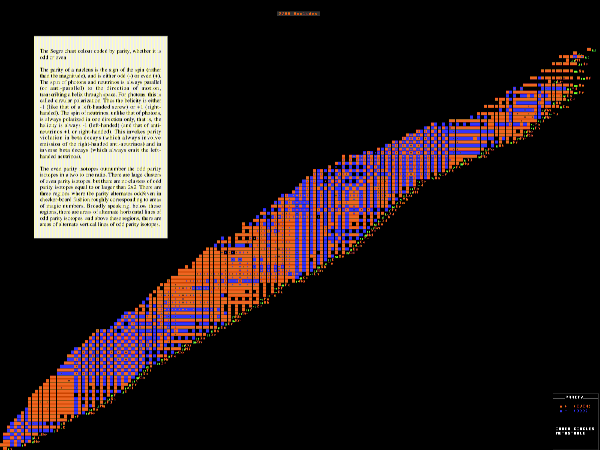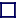### PARITY

The parity of a nucleus is the sign of the spin (rather than the magnitude), and is either odd (-) or even (+). Parity conservation is absolute except in the case of weak interactions (beta decay) where it is feebly broken. The spin of photons and neutrinos is always parallel (or anti-parallel) to the direction of motion, transcribing a helix through space. For photons, this is called circular polarization. Thus the helicity is either -1 (like that of a left-handed screw) or +1 (right-handed). In a magnetic field, the electron spin is similarly constrained to be either parallel or anti-parallel to the magnetic field. At cryogenic temperatures and in a strong magnetic field cobalt-60 nuclei should beta-decay emitting electrons with spin split 50:50 parallel/anti-parallel to the magnetic field. However, a slight preference for left-handed spin electrons was observed, thus breaking parity conservation. The spin of neutrinos, unlike that of photons, is always polarized in one direction only, that is, the helicity is always -1 (left-handed) (and that of anti-neutrinos +1 or right-handed). This invokes parity violation in beta decays (which always involve emission of the right-handed anti-neutrinos) and in inverse beta decays (which always emit the left-handed neutrinos), and in other weak-interaction processes. Parity violation is not well understood but may well have a bearing on the matter/anti-matter imbalance.Enlarge [POP]

Segre Chart Colour-coded by Parity
Red - even; blue - odd
The even parity nuclides easily outnumber the odd parity nuclides. Moreover, on the Segre chart, there are large clusters of even parity nuclides but there are no clusters of odd parity nuclides equal to or larger than 2x2. There are three regions where the parity alternates odd/even in checker-board fashion. Broadly speaking, below these regions, there are areas of alternate horizontal lines of odd parity nuclides, and above these regions, there are areas of alternate vertical lines of odd parity nuclides.

Parity is ultimately conserved except in beta decay. In decay chains, beta decay can alter the parity, but alpha decay should not (but there are examples in the data where it in-explicably does! Perhaps the explanation lies in the possibility that these nuclides decay to a metastable state that does have the correct parity [The Parity chart shows the parity of the ground-state, and by the inner circles the parity of any metastable-state] ).